# CVOL for Interest Rates: Understanding Volatility in Price and Yield Terms

• 10 Mar 2021
• By Eric Leininger and John Wiesner
• Topics: Interest Rates

The CME Group Volatility Index, or CVOL, offers a dual lens of price and yield volatility for interest rate products.

Uncertainty comes in many forms. Implied volatility, the implied standard deviation of price ranges derived from option prices, is a mathematical construct that market participants use to quantify uncertainty. CME Group CVOL indexes produce robust volatility estimates using the deep liquidity of its interest rate options markets. As with all CVOL Indexes, Treasury CVOL Indexes provide additional information about the volatility surface from four auxiliary indicators: UpVar, DnVar, Skew, and Convexity.

Importantly for fixed income participants, Treasury CVOL Indexes come in both yield and price volatility formats. Track yield volatility or futures price volatility to quickly capture market pricing of the same potential moves in either yield or price terms.

### Figure 1: 10-Year Treasury CVOL Indexes

Source: CME Group

With the interest rate environment so interesting and complex, and with continued consolidation of banking and trading activity across interest rate products, investors and traders need an advanced volatility tool to effectively manage interest rate uncertainty. This environment and these advancements to visualize implied volatility of yields allow comparisons of over-the-counter (OTC) products such as swaptions to exchange traded options and vice versa.

Effectively calculating a robust Treasury yield volatility requires two steps. First, calculate the implied volatility of the futures price, and then second, use the dollar value of a basis point (DV01) to transform the price volatility to an equivalent yield volatility.

To do this, CME Group first uses yield variance, the squared standard deviation, from its CVOL calculation.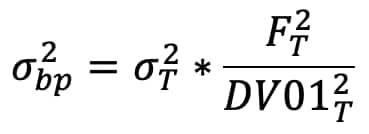is the variance calculation at a single tenor, ”T”,  for the future.

FT is the futures contract settlement price, DV01T is the first derivative with a respect to a 1 basis point change in yield. Here DV01T is taken from the relevant cheapest to deliver (CTD) of the comparable Treasury futures basket that corresponds to FT.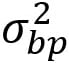is the CVOL variance of the yield.

The standard time weighting methodology for arriving at a specific time horizon, (typically H = 30 days), is employed to generate the implied standard deviation,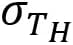, at time T = H.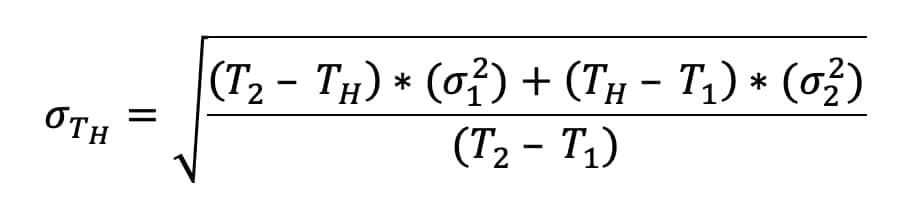This same formula is employed but with the corresponding DV01s substituted for the respective futures settlements,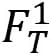&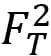. Substituting the DV01 formula to the time weighted interest rate price variance transforms it to interest rate yield variance. The square root is then taken to deliver interest rate yield volatility for time horizon T = H.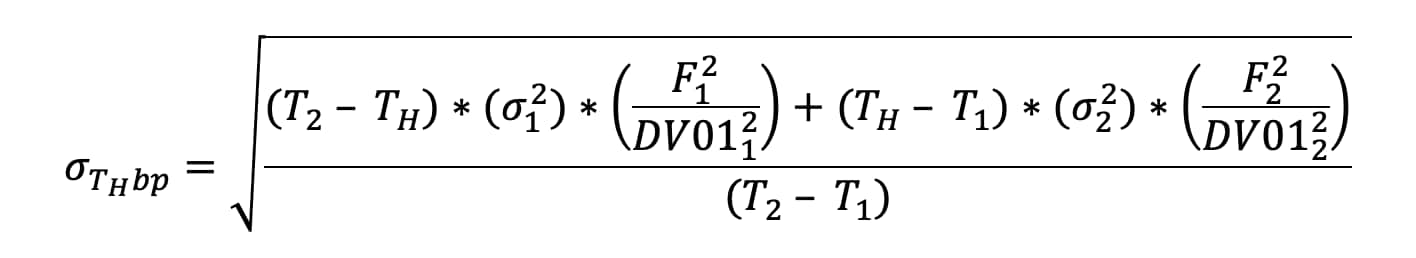The above transformations enable the dual price and yield versioning of the CVOL Index for interest rates. Now, by employing CVOL, market participants can easily visualize and use both price and yield volatility measurements.

## UpVar and DnVar

Included with the CVOL Index family are the supporting indicators, UpVar (up variance) and DnVar (down variance). They are calculated by using out-of-the-money calls for UpVar and out-of-the-money puts for DnVar, respectively.

“UpVar” is intended to convey the idea that the volatility metric is drawn from options that are struck at prices that would require the futures price to increase or rise up to be at any of those strikes. “DnVar” is calculated from options that are struck below the current futures price, hence a metric concerning prices at strikes that are lower than or down from the current price level.

The UpVar for the Treasury Price Volatility Index is drawn from options prices that are struck higher than the futures contract price, and the DnVar is drawn from puts below the futures contract price, as would be normally expected for a CVOL metric; but the yield version of both UpVar and DnVar must be understood inversely.

### Figure 2: TYH1 implied volatility curve

Source: Quikstrike

UpVar and DnVar need the same DV01-based conversion from price to yield that the regular CVOL metric requires, but they also require rearrangement.

“Up” in futures or bond prices means “down” in yield terms, and so the CVOL UpVar and DnVar for futures appropriately beg to be renamed when the price to yield transformation is applied.

The DnVar metric for the yield is ultimately predicated on futures call prices, and UpVar on futures put prices, which may seem counterintuitive, but the price-to-yield conversion requires this reframing of “up” and “down.”

Finally, CVOL Skew requires a similarly nuanced understanding between price and yield.

The CVOL family of indicators includes two versions of skew. The first version of skew is simply the numerical difference between the UpVar and DnVar, i.e. UpVar minus DnVar. The second version of skew is the ratio between UpVar and DnVar.

If DnVar is greater than UpVar, then the first skew metric would be a negative value, and the ratio version of skew would be less than 1.00. But when the conversion to yield CVOL happens, the sign would flip from negative to positive, and the ratio would be greater than 1.00.

In simpler terms, if the futures or bonds are expecting more downside risk, then the rate or yield itself would have more upside risk. Therefore, after the conversion from price terms to yield terms, the sign will flip for skew and the skew ratio will be inverted.

### Figure 3: 10Y Yield CVOL Index, skew, and underlying futures price

Source: CME Group, QuikStrike